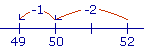S k i l l
i n
A R I T H M E T I C

Lesson 7

# THE MEANING OF SUBTRACTION

## Mental Calculation

First, we will see the meaning of subtraction. Then we will see methods for achieving it quickly.

IN ADDITION we are given two numbers and we have to name their sum.

28 + 7 = ?

But in what is called the inverse of addition, we are given the sum and we have to name one of the two numbers.

28 + ? = 35.

That has been called "subtraction ." It is the problem of naming the number we must add to 28 to get 35.

 28 + 7 = 35. 35 − 28 = 7.

The sign of subtraction is the horizontal dash (−), which we read "minus."

"35 minus 28 equals 7."

We say that the difference between 35 and 28 is 7.

 1. What is the problem of "subtraction"? Larger − Smaller = Difference. Smaller + Difference = Larger. Given two numbers, a Larger and a Smaller, we are to name the number we must add to the Smaller so that the sum will equal the Larger. That number we add is called the Difference of the two numbers. We say that we are subtracting the Smaller number from the Larger.

The historical name subtraction -- literally, "taken away from below" -- refers to a specific written method, namely writing the smaller number (the subtrahend) under the larger (the minuend), and doing the method. However, that is not the only way of finding the difference of two numbers. These days we could use a calculator! In any event, we should try to come up with a new name that emphasizes the meaning of the inverse of addition.

Although we use the name subtraction in this lesson, we do not mean the written method. We mean naming the number we must add to the smaller number.

When we subtract two numbers, we call them the terms.  Thus when we write 35 − 28, the terms are 35 and 28.  We also call '35 − 28' a difference—even if we do not name the answer.

If the terms are equal, of course, then their difference is 0.

5 − 5 = 0.

As in addition, we can only subtract quantities of the same kind, that is, that have the same name.

35 dollars − 28 dollars = 7 dollars.

Example 1.   Joan had \$14 and she spent \$9. How much does she have left? That is:  How much is 14 − 9?

9 + 5 = 14.14 − 9 = 5.

We can say that the difference of two numbers is the distance between them.  How far is it from 9 to 14?  A distance of 5.

Here is a simple example:

8 − 6 = 2  because  6 plus 2 = 8.

Knowing that, you would also know

 80 − 60 = 20   because 60 plus 20 is 80. 84 − 60 = 24   because 60 plus 24 is 84. 812 − 600 = 212   because 600 plus 212 is 812.

"Subtracting" is adding to the smaller number.  And the sum of the smaller number plus the difference, must equal the larger number.

Example 2.   Prove:  472 − 370 = 102.

Solution.   370 plus 102 = 472.

That is the meaning of subtraction. And therefore that is how to prove it.

Problem.   If you subtract 198 from 4,685, you will obain the difference 4,487.

4,685 − 198 = 4,487.

What difference will you obtain if you subtract 4,487 from 4,685, and why?

4,685 − 4,487 = ?

You will obtain 198.  Because 198 + 4,487 will always equal 4,685.

The complement to 10

The student should know all the ways of  "completing" 10.  For example,

10 − 3 = ?

3 plus what number makes 10?   7, of course.  7 is called the complement to 10 of 3.   7 "completes" 3 to make 10.

Example 3.   10 − 6 = 4.  In fact, whenever we take a 6 from any multiple of 10 -- 20, 30, 40, 50, 60 -- we always get a 4.

30 − 6 = 24

40 − 6 = 34

80 − 6 = 74

Similarly, each of the following differences will end in the complement to 10 of the smaller number.

50 − 8 = 42

70 − 9 = 61

200 − 3 = 197

\$8.00 − 4¢ = \$7.96

These are not the kind of problem to write in a column and do some written methodLet us now look at subtracting from a two-digit number.  We can think of it as "taking away" the smaller number.

50 − 1 = 49.

"50 take away 1 is 49."

60 − 2 = 58.

"60 take away 2 is 58."

70 − 3 = 67.

"70 take away 3 is 67."

 2. How can we subtract a one-digit number from a two-digit number, mentally? 52 − 3Break off from the smaller number the ones digit of the larger (2), and subtract to get a multiple of 10. 52 − 2 = 50. Then subtract the rest of the smaller number. 50 − 1 = 49.

Example 4.   Calculate mentally  83 − 5.

Solution.   "83 minus 3 is 80; minus 2 is 78."

Example 5.   Calculate mentally  72 − 6.

Solution.   "72 minus 2 is 70; minus 4 is 66."

Example 6.   A book has 195 pages, and you have read 7 of them. How many pages are left?

Answer.   "195 minus 5 is 190; minus 2 is 188."

At this point, please "turn" the page and do some Problems.

or

Continue on to the next Section.